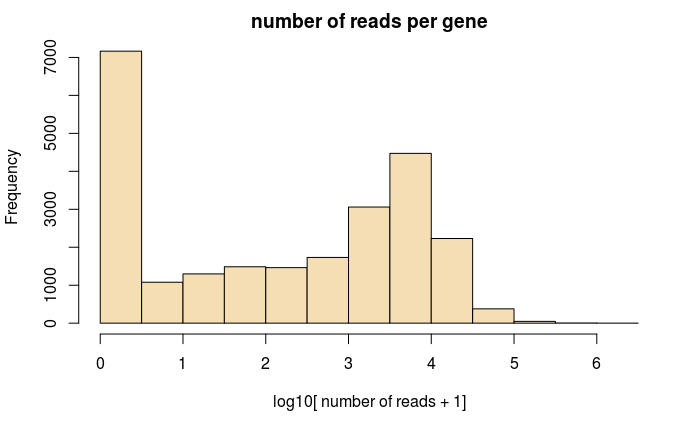The notebook shows anlaysis of a SMART-seq2 dataset, where we start with per-cell bam files. It shows how to estimate gene-relative velocity (with different pooling options), as well as how to estimate gene-relative velocity.

``library(velocyto.R)``

Load the data and genome annotations:

(this block will note evalaute by default, as it takes a while)

``````# if you want to run this, please download bam files (and genes.refFlat) from
# http://pklab.med.harvard.edu/velocyto/chromaffin/bams.tar
# and extract it ("tar xvf bams.tar") in the working directory.
# note: the file is fairly large - 5.6 GB!
path <- "data/e12.5.bams"
files <- system(paste('find',path,'-name "*unique.bam" -print'),intern=T)
names(files) <- gsub(".*\\/(.*)_unique.bam","\\1",files)
# parse gene annotation, annotate bam file reads

``dat <- readRDS(url("http://pklab.med.harvard.edu/velocyto/chromaffin/dat.rds"))``

Read in cell cluster assignment and tSNE embedding used in the Furlan et al. (Science’17).

``````cell.colors <- readRDS(url("http://pklab.med.harvard.edu/velocyto/chromaffin/cell.colors.rds"))

## Gene filtering

Spliced expression magnitude distribution across genes:

``hist(log10(rowSums(dat\$emat)+1),col='wheat',xlab='log10[ number of reads + 1]',main='number of reads per gene')``Set up expression matrices, filtering genes to leave those that exceed some pre-defined g to the average expression magnitude

``````# exonic read (spliced) expression matrix
emat <- dat\$emat;
# intronic read (unspliced) expression matrix
nmat <- dat\$iomat;
# spanning read (intron+exon) expression matrix
smat <- dat\$smat;
# filter expression matrices based on some minimum max-cluster averages
emat <- filter.genes.by.cluster.expression(emat,cell.colors,min.max.cluster.average = 5)
nmat <- filter.genes.by.cluster.expression(nmat,cell.colors,min.max.cluster.average = 1)
smat <- filter.genes.by.cluster.expression(smat,cell.colors,min.max.cluster.average = 0.5)
# look at the resulting gene set
str(intersect(intersect(rownames(emat),rownames(nmat)),rownames(smat)))``````
`` chr [1:5920] "Dmd" "Rbfox1" "Dlg2" "Auts2" "Ctnna2" "Rbms3" "Gpc6" "Pard3b" "Dpyd" "Hdac9" "Celf2" "Erc2" ...``

## Several variants of velocity estimates using gene-relative model

We’ll start with what is perhaps the most robust estimate, that combines cell kNN pooling with the gamma fit based on an extreme quantiles:

Using min/max quantile fit, in which case gene-specific offsets do not require spanning read (smat) fit. Here the fit is based on the top/bottom 2% of cells (by spliced expression magnitude)

``rvel.qf <- gene.relative.velocity.estimates(emat,nmat,deltaT=1,kCells = 5,fit.quantile = 0.02)``
``````calculating cell knn ... done
calculating convolved matrices ... done
fitting gamma coefficients ... done. succesfful fit for 8646 genes
filtered out 1335 out of 8646 genes due to low nmat-emat correlation
filtered out 968 out of 7311 genes due to low nmat-emat slope
calculating RNA velocity shift ... done
calculating extrapolated cell state ... done``````

We visualize the velocities by projecting observed and extrapolated cells onto the first 5 PCs:

``pca.velocity.plot(rvel.qf,nPcs=5,plot.cols=2,cell.colors=ac(cell.colors,alpha=0.7),cex=1.2,pcount=0.1,pc.multipliers=c(1,-1,-1,-1,-1))``
``log ... pca ... pc multipliers ... delta norm ... done``